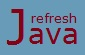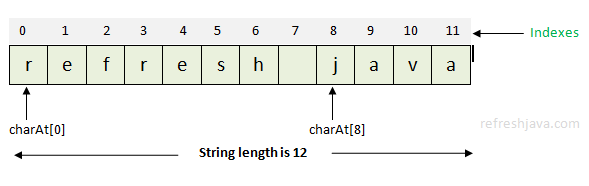# Useful String Class Methods in Java

Sometimes a programmer may need to perform some types of operations on strings like "compare two strings, find or replace a particular character/word in a string, add two strings, extract a part of a given string" etc. Considering these needs in mind java designer has given number of methods in `String` class which you can use in your program to perform such type of operations on strings.

To understand the `String` class methods better, let's first understand how a string value in java is stored in memory. String in java is an array of characters, so string values in java is stored as an array of characters where each characters have an index number. Some of the `String` class methods uses these indexes to perform the operations on string. The image below shows how a string value `"refresh java"` will be stored as an array of characters in string pool memory.As you can see each characters have been given an index number and you can access the character at a given index using `charAt()` method of `String` class which returns the character at given index. The length of a string is the total number of characters in string. To understand the `String` class methods better let's consider we have three string variables `s1, s2` and `s3` as given below.

``` String s1 = `"refresh java"`;
String s2 = `"Hello World"`;
String s3 = `"learn java on refresh java tutorial"`;```

### How can I call the method of String class in my program ?

To access any method of `String` class you just have to use dot(`.`) operator with string variable or string literal, for example ```s1.length(), s1.equals(s2), "Hello".equalsIgnoreCase("hello"), s1.chartAt(8)``` etc.

Table below shows the list of methods which are used often with string. You can refer oracle documentation of `String` class to get the detail of all methods.

Name Description Example
`int` length() It returns the length of string. The length is equal to the total number of characters in string. `int` length = s1.length();
`//length = 12`
`boolean` equals(Object anObject) Used to compare two strings. It returns `true` if both string represents same sequence of characters else `false`. s1.equals(s2); ` // returns false`

s1.equals(`"refresh java"`);
` // returns true`.
`boolean` equalsIgnoreCase(String anotherString) Used to compare two strings, ignoring the case(upper or lower case). It returns `true` if both the string are of same length and represents same sequence of character ignoring the case else returns `false`. s1.equalsIgnoreCase(`"Refresh JAVA"`);
`// returns true`
`boolean` startsWith(String prefix) checks if a string starts with the string represented by `prefix`. s1.startsWith(`"refresh"`);
`// returns true`.

s1.startsWith(`"Refresh"`);
`// returns false.`
`int` indexOf(String str) Used to search if a substring(represented by `str`) exist in the given string. Returns the index of first occurrence of substring in the given string if found else returns -1. s1.indexOf(`"java"`);
`// returns 8`

s1.indexOf(`"hello"`);
`// returns -1`
`int` indexOf(String str, `int` fromIndex) Used to search if substring(represented by `str`) exist in the given string, but it starts searching from the index given by fromIndex. It returns starting index of that substring if found else returns -1. s3.indexOf(`"java"`, 10);
`// returns 22`

s3.indexOf(`"easy"`, 25);
`// returns -1`
`int` lastIndexOf(String str) Used to search the last occurrence of substring(represented by `str`) in the given string. It returns the index of last occurrence of substring if found else returns as -1. s3.lastIndexOf(`"java"`);
`// returns 22`
`char` charAt(`int` index) Returns the character at specified `index` in given string. `char` charVar = s1.charAt(8);
`// charVar = 'j'`
String substring(`int` fromIndex) Returns a string that is a substring of the given string. The substring starts with the character at `fromIndex` position and extends to the end of given string. String str = s1.substring(8);
`// str = "java", s1 will still be same that is "refresh java"`

String str2 = s3.substring(27);
`// str2 = "tutorial", s3 will still be same.`
String substring(`int` fromIndex, `int` endIndex) Returns a string that is a substring of the given string. The substring starts with the character at `fromIndex` position and extends to the `(endIndex-1)` String str = s1.substring(0,7);
`// Return characters from 0 to 6 str = "refresh"`

String str2 = s3.substring(14,26);
`// str2 = "refresh java"`
String toLowerCase() Converts all the characters of given string to lower case and returns that string. String str = s2.toLowerCase();
`// str = "hello world" s2 will still be same that is "Hello World".`
String toUpperCase() Converts all the characters of given string to upper case and returns that string. String str = s1.toUpperCase();
`// str = "REFRESH JAVA", s1 will still be same.`
String trim() Used to remove whitespace( ) at the beginning and end (not in between) of a given string. Returns a string without any whitespace in end and beginning. String str = `" hello world "`.trim();
`// str = "hello world"`
String concat(String str) Concatenates(adds) the string represented by `str` to the end of given string String strVar = s1.concat(`" tutorial"`);
`// strVar = "refresh java tutorial", s1 will still be same.`
`char`[] toCharArray() Converts the given string to a new character array and returns that char array. `char` [] charArray = s1.toCharArray();
```// charArray will contain an array of characters(r,e,f,r,e,s,h, ,j,a,v,a)```
String replace(`char` oldChar, `char` newChar) Returns a string resulting from replacing all occurrences of character represented by `oldChar` with character represented by `newChar` in the given string. String str = s1.replace(`'r'`, `'h'`);
```// str = "hefhesh java", s1 will still be same.```
String replaceFirst(String regexe, String replacement) Used to replace first occurrence of string represented by `regexe` with string represented by `replacement` in given string. `regexe` can be a regular expression as well. String str = s3.replaceFirst(`"java"`,`"program"`);
```// str = "learn program on refresh java tutorial"```
String replaceAll(String regexe, String replacement) Used to replace all occurrence of string represented by `regexe` with string represented by `replacement` in given string, `regexe` can be a regular expression as well. String str = s3.replaceAll(`"java"`,`"program"`);
```// str = "learn program on refresh program tutorial"```
String[] split(String regexe) Used to split a given string on the basis of `regexe`. It returns a string array. String[] str = s3.split(`" "`);
```// str will contains strings "learn" "java" "on" "refresh" "java" "tutorial"```

### String class methods program in Java

```` class` StringClassMethods
{
`public static void` main(String [] args)
{
String s1 = `"refresh java";`
String s2 = `"Hello World";`
String s3 = `"learn java on refresh java tutorial";`
String str;

`// Getting the length of a string`
System.out.println(`"length of string s1 = "`+s1.length());
`// Comparing two strings`
System.out.println(s1.equals(`"refresh java"`));
System.out.println(`"is s1 equals s2 = "`+s1.equals(s2));
`// Checking if a string starts with a given substring`
System.out.println(`"is s1 starts with word refresh = "`+s1.startsWith(`"refresh"`));
`// Searching a substring in a string`
System.out.println(`"index of word java in s3 = "`+s3.indexOf(`"java"`));
System.out.println(`"index of word hello in s3 = "`+s3.indexOf(`"hello"`));
`// Getting the character at given index in a string`
System.out.println(`"character at index 8 in string s1 = "`+s1.charAt(8));
`// Extracting a substring from a given string`
str = s1.substring(8);
System.out.println(`"Substring str = "`+str);
`// After extracting a substring, value of s1 will still remain same.`
System.out.println(`"Value of s1 = "`+s1);
`// Converting a given string to lower case.`
str = s2.toLowerCase();
System.out.println(`"Lower case str = "`+str);
`// Concatenating a string to given string.`
str = s1.concat(`" tutorial"`);
System.out.println(`"Concatenated str = "`+str);
`// Concatenating a string to given string using + operator.`
str = str + `" online"`;
System.out.println(`"+ operated value of str = "`+str);
`// Replacing a word in a given string.`
str = s3.replaceFirst(`"java"`,`"program"`);
System.out.println(`"Replaced value str = "`+str);
`// Splitting a given string using space( ).`
String[] strArray = s3.split(`" "`);
`for`(String strVar : strArray)
System.out.print(strVar+`", "`);
}
}```

Output:

length of string s1 = 12
true
is s1 equals s2 = false
is s1 starts with word refresh = true
index of word java in s3 = 6
index of word hello in s3 = -1
character at index 8 in string s1 = j
Substring str = java
Value of s1 = refresh java
Lower case str = hello world
Concatenated str = refresh java tutorial
+ operated value of str = refresh java tutorial online
Replaced value str = learn program on refresh java tutorial
learn, java, on, refresh, java, tutorial,

## Difference between equals() and == in Java

Both equals() method and == operator can be used to compare string variables or string literals in java but there is a difference between both. equals() method compares the content(the sequence of characters) of string variables or string literals. It returns `true` if both the content are same else returns `false` while == operator compares the references(address) of string variables or string literals. It returns `true` if both the variables or literals points to same reference else returns `false`.

If the comparison of two strings using == operator returns `true` then equals() method for same comparison will definitely return `true` while vice-versa may or may not return `true`. The program given below shows the difference of equals() method and == operator.

```` class` StringComparison
{
`public static void` main(String [] args)
{
String str = `"refresh java"`;
String str2 = new String(`"refresh java"`);
String str3 = `"refresh java"`;
String str4 = new String(`"refresh java"`);
String str5 = str2;

System.out.println(`"Comparison using equals() method .........."`);
System.out.println(`"str equals() str2 >> "`+str.equals(str2));
System.out.println(`"str equals() str5 >> "`+str.equals(str5));
System.out.println(`"str2 equals() str4 >> "`+str2.equals(str4));
System.out.println(`"str2 equals() str5 >> "`+str2.equals(str5));

System.out.println(`"Comparison using == operator .........."`);
System.out.println(`"str == str2 >> "`+(str == str2));
System.out.println(`"str == str3 >> "`+(str == str3));
System.out.println(`"str2 == str4 >> "`+(str2 == str4));
System.out.println(`"str2 == str5 >> "`+(str2 == str5));
System.out.println(`"str4 == str5 >> "`+(str4 == str5));
}
}```

Output:

Comparison using equals() method ..........
str equals() str2 >> true
str equals() str5 >> true
str2 equals() str4 >> true
str2 equals() str5 >> true
Comparison using == operator ..........
str == str2 >> false
str == str3 >> true
str2 == str4 >> false
str2 == str5 >> true
str4 == str5 >> false

★★★
• String methods whose return type is String, always returns a new string, the original string still remains same in string pool.
• String.valueOf() method can be used to convert any primitive data type value as string eg. String.valueOf(10), String.valueOf(20.5) etc.
• Integer values represented as string can be converted to integer using Integer.parseInt() method, eg. Integer.parseInt("10").
• Ensure that your string variable is not null while calling a string method, otherwise your program will throw NullPointerExcetpion at runtime.